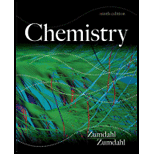# Impure nickel, refined by smelting sulfide ores in a blast furnace, can be converted into metal from 99.90% to 99.99% purity by the Mond process. The primary reaction involved in the Mond process is Ni ( s ) + 4CO ( g ) ⇌ Ni ( CO ) 4 ( g ) a. Without referring to Appendix 4, predict the sign of ∆S° for the above reaction. Explain. b. The spontaneity of the above reaction is temperature-dependent. Predict the sign of ∆S surr , for this reaction. Explain c . For Ni(CO) 4 ( g ), Δ H f o = − 607 KJ/mol and S ° = 417 J/K ·mol at 298 K. Using these values and data in Appendix 4, calculate ∆H° and ∆S° for the above reaction. d. Calculate the temperature at which ∆ G ° = 0 (K = 1) for the above reaction, assuming that ∆H° and ∆S° do not depend on temperature. e. The first step of the Mood process involves equilibrating impure nickel with CO( g ) and Ni(CO) 4 ( g ) at about 50°C. The purpose of this step is to convert as much nickel as possible into the gas phase. Calculate the equilibrium constant for the above reaction at 50.°C. f. In the second step of the Mood process, the gaseous Ni(CO) 4 is isolated and heated to 227°C. The purpose of this step is to deposit as much nickel as possible as pure solid (the reverse of the preceding reaction). Calculate the equilibrium constant for the preceding reaction at 227°C. g. Why is temperature increased for the second step of the Mood process? h. The Mond process relies on the volatility of Ni(CO) 4 for its success. Only pressures and temperatures at which Ni(CO) 4 is a gas are useful. A recently developed variation of the Mood process carries out the first step at higher pressures and a temperature of l52°C. Estimate the maximum pressure of Ni(CO) 4 (g) that can be attained before the gas will liquefy at 152°C. The boiling point for Ni(CO) 4 is 42°C and the enthalpy of vaporization is 29.0 kJ/mol. [Hint: The phase change reaction and the corresponding equilibrium expression are Ni ( CO ) 4 ( l ) ⇌ Ni ( CO ) 4 ( g ) K = P Ni ( CO ) 4 Ni(CO) 4 ( g ) will liquefy when the pressure of Ni(CO) 4 is greater than the K value.]### Chemistry

9th Edition
Steven S. Zumdahl
Publisher: Cengage Learning
ISBN: 9781133611097### Chemistry

9th Edition
Steven S. Zumdahl
Publisher: Cengage Learning
ISBN: 9781133611097

#### Solutions

Chapter
Section
Chapter 17, Problem 119MP
Textbook Problem

## Expert Solution

### Want to see the full answer?

Check out a sample textbook solution.See solution

### Want to see this answer and more?

Experts are waiting 24/7 to provide step-by-step solutions in as fast as 30 minutes!*

See Solution

*Response times vary by subject and question complexity. Median response time is 34 minutes and may be longer for new subjects.